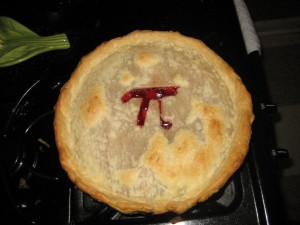# Pi DayWhen the number Pi (π) is expressed as a decimal, it’s written as 3.14. So we came up with Pi Day, which we celebrate on the 14th day of the 3rd month every year (3/14). Really it’s just an excuse for kids at school to eat pie during their math lesson.

This year’s Pi Day is a little more exciting though. The number Pi isn’t just 3.14. It’s decimal expansion is never ending. And the next two numbers are 1 and 5. So this year, 2015, the date lines up perfectly. Pi is 3.1415 and Pi Day will be 3/14/15.

If you want to get really crazy you can celebrate on March 14, 2015, at 9:26.53 a.m. since the next numbers are 9, 2, 6, 5, and 3. (We might see the same fuss next year because if you round off Pi to four digits, it’s actually 3.1416 instead of 3.1415).

The holiday might be silly, but the number itself is really fascinating.

Pi is the ratio between the circumference of a circle and the diameter of a circle. The ratio is exactly the same no matter the size of the circle.

There’s actually no fraction that exactly equals Pi. While 22/7 is close, and 355/113 is even closer, Pi’s decimal expansion goes on forever. We can now compute trillions of decimal digits for Pi, but there will never be a simple mathematical way to express it with that much precision.

The number also shows up in a lot of unexpected places. If you pick two numbers at random, the probability that they won’t have any common factors is 6/π². It shows up in the measurements of the Great Pyramid of Giza. It shows up in the angular distance between stars. Pi shows up in literally thousands of equations.

It’s such a fundamental part of math and science, but impossible to completely pin down because its decimal places march on into infinity. It’s incredible that Pi describes something so simple — the circle — but unravels into a value that is mind blowingly complex.

Posted in Education, Events, Holidays, News, World.